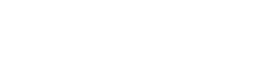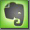Common Mode Rejection

Common Mode Voltage and Common Mode Rejection (CMR)

In a balanced audio line, any voltage that appears on both conductors (inputs), could be cancelled (in an ideal case only!). The unwanted signal voltage is COMMON to both inputs, it is a COMMON-MODE voltage. Because the differential amplifier of the balanced line responds only to the differential voltage between the two conductors, the common-mode voltage is cancelled out.
See Balanced Lines.

Common Mode Rejection is the ability of a balanced (or differential) input to reject the part of the incoming signal which has the same amplitude and phase on both input terminals, referenced to ground (The ability to respond to only differences at the input terminals).
The original audio signal has a positive part on one termination and a negative part (opposite polarity) on the other. Any signal 'common' to both terminals will eventually get rejected, cancelled at the receiving end.A differential amplifier rejects any common-mode noise at its input by a factor equal to its common-mode rejection ratio (normally over 1000:1). Input transformers could have a common mode rejection ratio of up to 250,000 : 1. The balanced audio transformer introduces a higher common mode rejection ratio than any other electric circuit. Noise immunity of balanced audio lines is dependant on how well the in- and output impedances are balanced and especially the common mode rejection ratio of the audio input channel.

Common Mode Rejection Ratio (CMRR)

The common mode rejection ratio (CMRR) is the ratio of Common Mode Noise (CMN) rejected and thereby prevented from converting to 'normal mode' voltage. CMRR is specified in dB. A ratio of 10 between two voltages equals 20dB. If a line is perfectly balanced, the common mode voltages cancel out completely. But this is not possible in real nature by 100%. The actual degree of cancellation is called the common mode rejection ratio.